SNAP Library 6.0, Developer Reference  2020-12-09 16:24:20 SNAP, a general purpose, high performance system for analysis and manipulation of large networks
TRowIterator Class Reference

Iterator class for TTable rows. More...

`#include <table.h>`

Collaboration diagram for TRowIterator: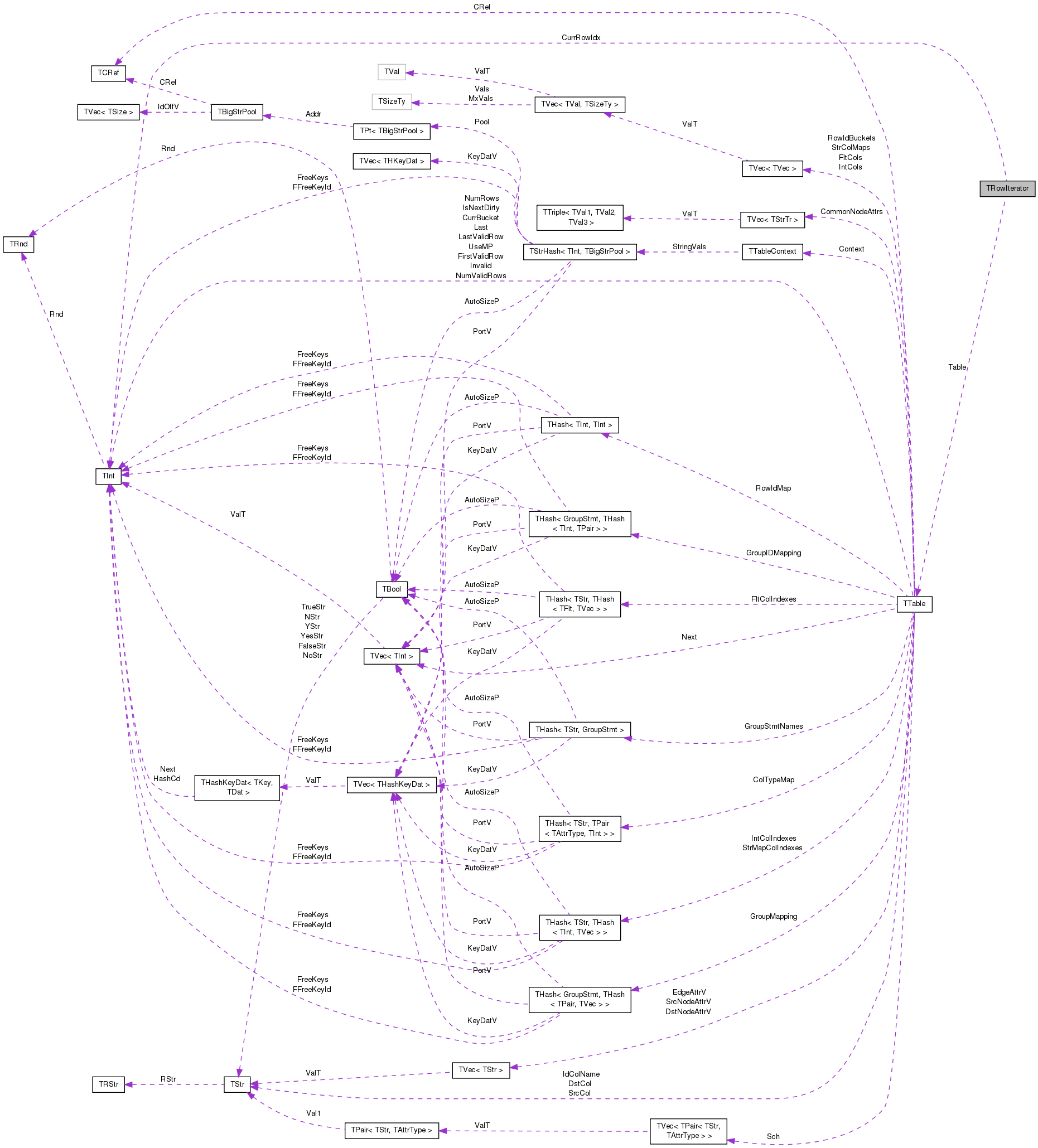[legend]

## Public Member Functions

TRowIterator ()
Default constructor. More...

TRowIterator (TInt RowIdx, const TTable *TablePtr)
Constructs iterator to row `RowIds` of `TablePtr`. More...

TRowIterator (const TRowIterator &RowI)
Copy constructor. More...

TRowIteratoroperator++ (int)
Increments the iterator. More...

TRowIteratorNext ()
Increments the iterator (For Python compatibility). More...

bool operator< (const TRowIterator &RowI) const
Checks if this iterator points to a row that is before the one pointed by `RowI`. More...

bool operator== (const TRowIterator &RowI) const
Checks if this iterator points to the same row pointed by `RowI`. More...

TInt GetRowIdx () const
Gets the id of the row pointed by this iterator. More...

TInt GetIntAttr (TInt ColIdx) const
Returns value of integer attribute specified by integer column index for current row. More...

TFlt GetFltAttr (TInt ColIdx) const
Returns value of floating point attribute specified by float column index for current row. More...

TStr GetStrAttr (TInt ColIdx) const
Returns value of string attribute specified by string column index for current row. More...

TInt GetStrMapById (TInt ColIdx) const
Returns integer mapping of a string attribute value specified by string column index for current row. More...

TInt GetIntAttr (const TStr &Col) const
Returns value of integer attribute specified by attribute name for current row. More...

TFlt GetFltAttr (const TStr &Col) const
Returns value of float attribute specified by attribute name for current row. More...

TStr GetStrAttr (const TStr &Col) const
Returns value of string attribute specified by attribute name for current row. More...

TInt GetStrMapByName (const TStr &Col) const
Returns integer mapping of string attribute specified by attribute name for current row. More...

TBool CompareAtomicConst (TInt ColIdx, const TPrimitive &Val, TPredComp Cmp)
Compares value in column `ColIdx` with given primitive `Val`. More...

TBool CompareAtomicConstTStr (TInt ColIdx, const TStr &Val, TPredComp Cmp)
Compares value in column `ColIdx` with given TStr `Val`. More...

## Private Attributes

TInt CurrRowIdx
Physical row index of current row pointed by iterator. More...

const TTableTable
Reference to table containing this row. More...

## Detailed Description

Iterator class for TTable rows.

An iterator class to iterate over all currently existing rows. Iteration over the rows should be done using only this iterator.

Definition at line 330 of file table.h.

## Constructor & Destructor Documentation

 TRowIterator::TRowIterator ( )
inline

Default constructor.

Definition at line 335 of file table.h.

335 : CurrRowIdx(0), Table(NULL) {}
const TTable * Table
Reference to table containing this row.
Definition: table.h:332
TInt CurrRowIdx
Physical row index of current row pointed by iterator.
Definition: table.h:331
 TRowIterator::TRowIterator ( TInt RowIdx, const TTable * TablePtr )
inline

Constructs iterator to row `RowIds` of `TablePtr`.

Definition at line 337 of file table.h.

337 : CurrRowIdx(RowIdx), Table(TablePtr) {}
const TTable * Table
Reference to table containing this row.
Definition: table.h:332
TInt CurrRowIdx
Physical row index of current row pointed by iterator.
Definition: table.h:331
 TRowIterator::TRowIterator ( const TRowIterator & RowI )
inline

Copy constructor.

Definition at line 339 of file table.h.

339 : CurrRowIdx(RowI.CurrRowIdx), Table(RowI.Table) {}
const TTable * Table
Reference to table containing this row.
Definition: table.h:332
TInt CurrRowIdx
Physical row index of current row pointed by iterator.
Definition: table.h:331

## Member Function Documentation

 TBool TRowIterator::CompareAtomicConst ( TInt ColIdx, const TPrimitive & Val, TPredComp Cmp )

Compares value in column `ColIdx` with given primitive `Val`.

Definition at line 190 of file table.cpp.

Referenced by TTable::SelectAtomicConst().

190  {
191  TBool Result;
192  switch (Val.GetType()) {
193  case atInt:
194  Result = TPredicate::EvalAtom(GetIntAttr(ColIdx), Val.GetInt(), Cmp);
195  break;
196  case atFlt:
197  Result = TPredicate::EvalAtom(GetFltAttr(ColIdx), Val.GetFlt(), Cmp);
198  break;
199  case atStr:
200  Result = TPredicate::EvalStrAtom(GetStrAttr(ColIdx), Val.GetStr(), Cmp);
201  break;
202  default:
203  Result = TBool(false);
204  }
205  return Result;
206 }
TFlt GetFltAttr(TInt ColIdx) const
Returns value of floating point attribute specified by float column index for current row...
Definition: table.cpp:159
TInt GetIntAttr(TInt ColIdx) const
Returns value of integer attribute specified by integer column index for current row.
Definition: table.cpp:155
TStr GetStrAttr(TInt ColIdx) const
Returns value of string attribute specified by string column index for current row.
Definition: table.cpp:163
static TBool EvalStrAtom(const TStr &Val1, const TStr &Val2, TPredComp Cmp)
Compare atomic string values Val1 and Val2 using predicate Cmp.
Definition: table.h:123
Definition: gbase.h:23
TStr GetStr() const
Definition: table.h:228
TInt GetInt() const
Definition: table.h:226
TAttrType GetType() const
Definition: table.h:229
Definition: gbase.h:23
static TBool EvalAtom(T Val1, T Val2, TPredComp Cmp)
Compare atomic values Val1 and Val2 using predicate Cmp.
Definition: table.h:110
bool Cmp(const int &RelOp, const TRec &Rec1, const TRec &Rec2)
Definition: bd.h:426
Definition: gbase.h:23
Definition: dt.h:974
TFlt GetFlt() const
Definition: table.h:227

Here is the call graph for this function: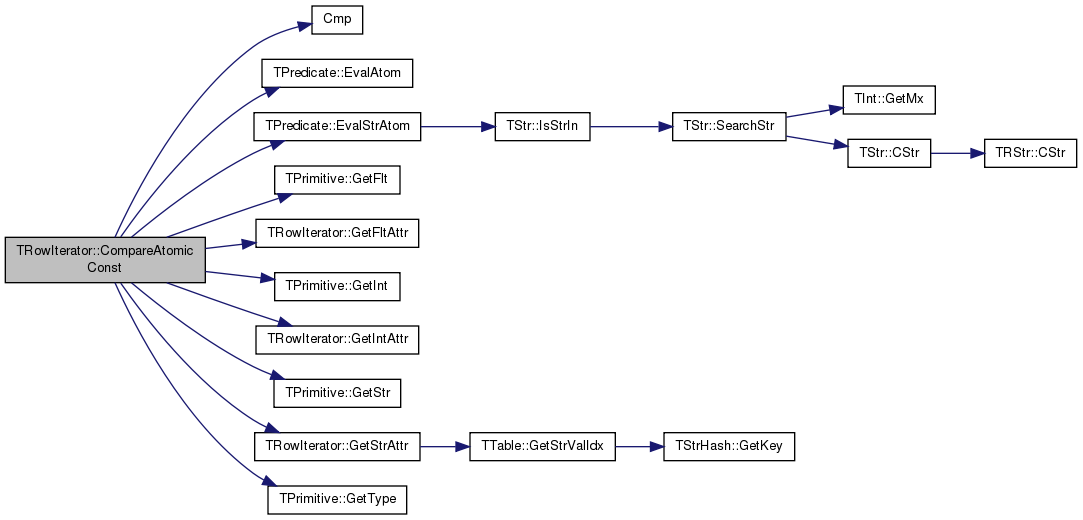Here is the caller graph for this function: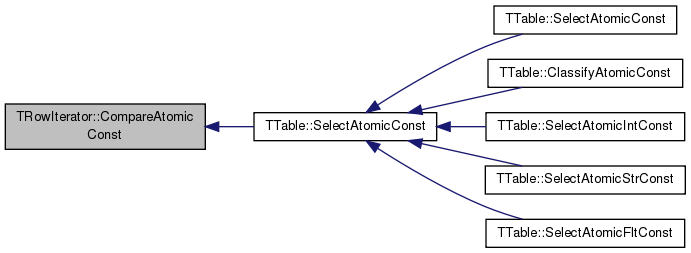TBool TRowIterator::CompareAtomicConstTStr ( TInt ColIdx, const TStr & Val, TPredComp Cmp )

Compares value in column `ColIdx` with given TStr `Val`.

Definition at line 208 of file table.cpp.

References TPredicate::EvalStrAtom(), and GetStrAttr().

Referenced by TTable::SelectAtomicConst().

208  {
209  TBool Result;
210  //printf("string compare\n");
211  Result = TPredicate::EvalStrAtom(GetStrAttr(ColIdx), Val, Cmp);
212  return Result;
213 }
TStr GetStrAttr(TInt ColIdx) const
Returns value of string attribute specified by string column index for current row.
Definition: table.cpp:163
static TBool EvalStrAtom(const TStr &Val1, const TStr &Val2, TPredComp Cmp)
Compare atomic string values Val1 and Val2 using predicate Cmp.
Definition: table.h:123
bool Cmp(const int &RelOp, const TRec &Rec1, const TRec &Rec2)
Definition: bd.h:426
Definition: dt.h:974

Here is the call graph for this function: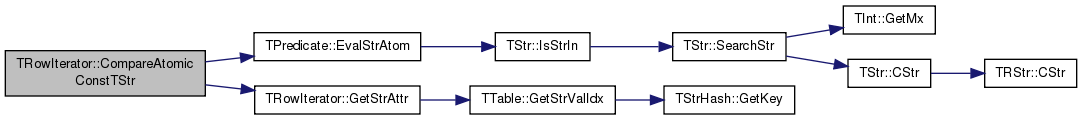Here is the caller graph for this function: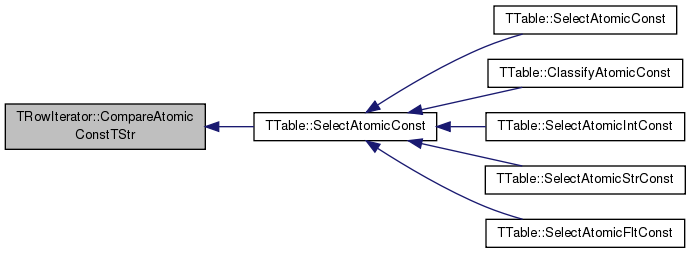TFlt TRowIterator::GetFltAttr ( TInt ColIdx ) const

Returns value of floating point attribute specified by float column index for current row.

Definition at line 159 of file table.cpp.

References CurrRowIdx, TTable::FltCols, and Table.

159  {
160  return Table->FltCols[ColIdx][CurrRowIdx];
161 }
const TTable * Table
Reference to table containing this row.
Definition: table.h:332
TVec< TFltV > FltCols
Data columns of floating point attributes.
Definition: table.h:559
TInt CurrRowIdx
Physical row index of current row pointed by iterator.
Definition: table.h:331

Here is the caller graph for this function: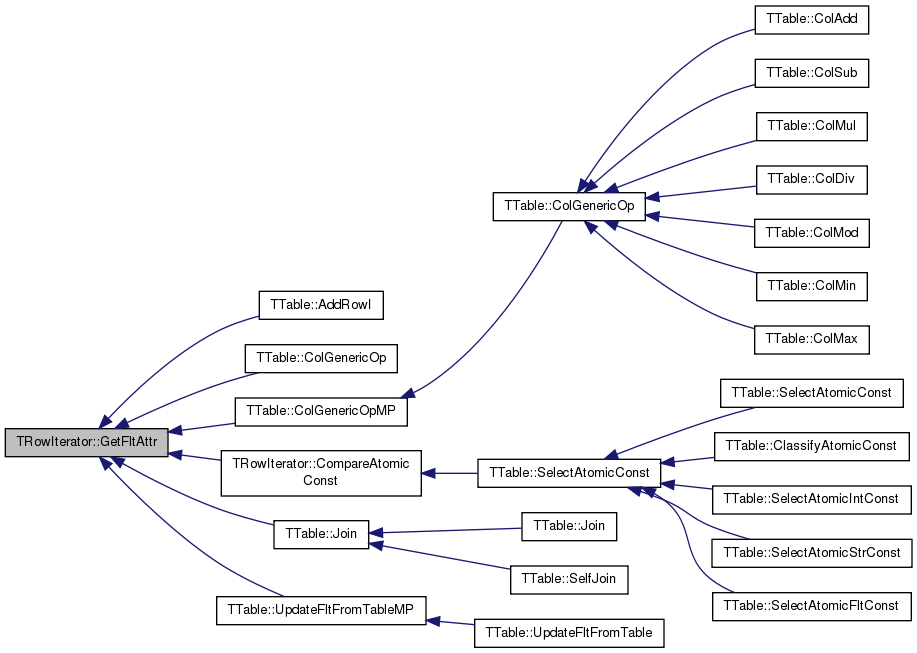TFlt TRowIterator::GetFltAttr ( const TStr & Col ) const

Returns value of float attribute specified by attribute name for current row.

Definition at line 172 of file table.cpp.

References CurrRowIdx, TTable::FltCols, TTable::GetColIdx(), and Table.

172  {
173  TInt ColIdx = Table->GetColIdx(Col);
174  return Table->FltCols[ColIdx][CurrRowIdx];
175 }
TInt GetColIdx(const TStr &ColName) const
Gets index of column ColName among columns of the same type in the schema.
Definition: table.h:1013
const TTable * Table
Reference to table containing this row.
Definition: table.h:332
Definition: dt.h:1137
TVec< TFltV > FltCols
Data columns of floating point attributes.
Definition: table.h:559
TInt CurrRowIdx
Physical row index of current row pointed by iterator.
Definition: table.h:331

Here is the call graph for this function: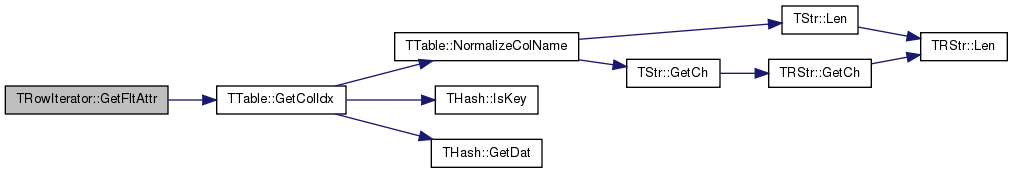TInt TRowIterator::GetIntAttr ( TInt ColIdx ) const

Returns value of integer attribute specified by integer column index for current row.

Definition at line 155 of file table.cpp.

References CurrRowIdx, TTable::IntCols, and Table.

155  {
156  return Table->IntCols[ColIdx][CurrRowIdx];
157 }
TVec< TIntV > IntCols
Next[i] is the successor of row i. Table iterators follow the order dictated by Next ...
Definition: table.h:558
const TTable * Table
Reference to table containing this row.
Definition: table.h:332
TInt CurrRowIdx
Physical row index of current row pointed by iterator.
Definition: table.h:331

Here is the caller graph for this function: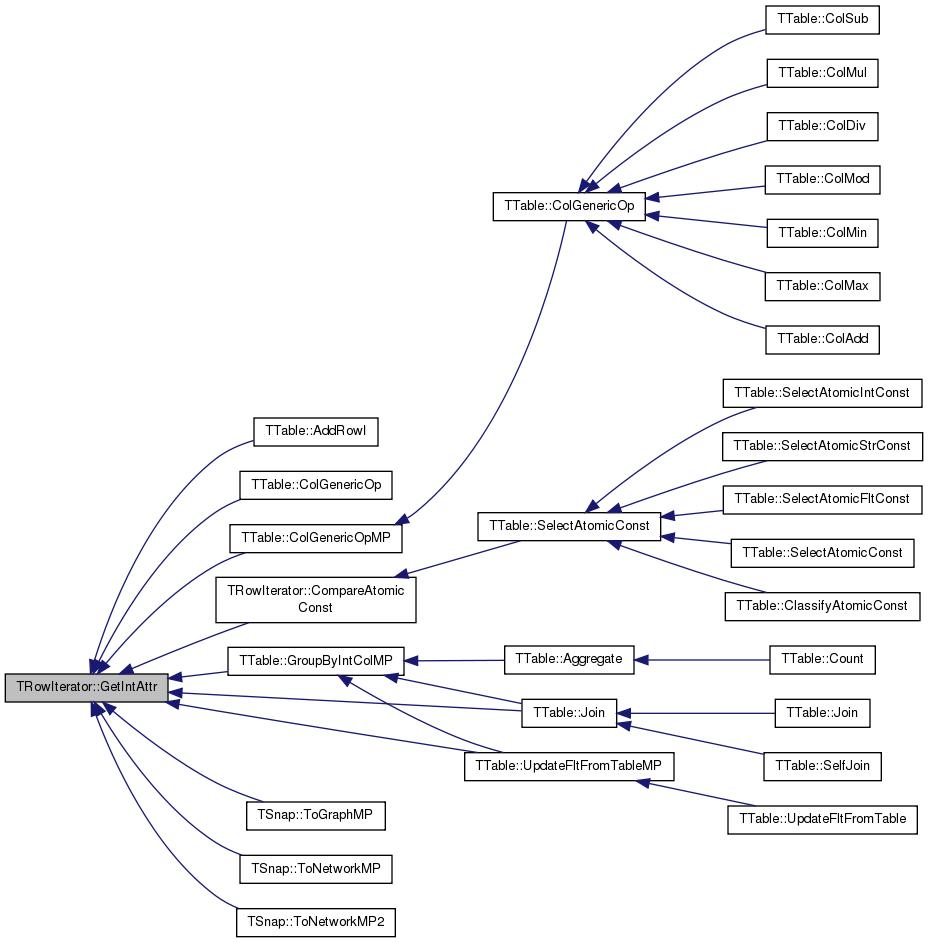TInt TRowIterator::GetIntAttr ( const TStr & Col ) const

Returns value of integer attribute specified by attribute name for current row.

Definition at line 167 of file table.cpp.

References CurrRowIdx, TTable::GetColIdx(), TTable::IntCols, and Table.

167  {
168  TInt ColIdx = Table->GetColIdx(Col);
169  return Table->IntCols[ColIdx][CurrRowIdx];
170 }
TInt GetColIdx(const TStr &ColName) const
Gets index of column ColName among columns of the same type in the schema.
Definition: table.h:1013
TVec< TIntV > IntCols
Next[i] is the successor of row i. Table iterators follow the order dictated by Next ...
Definition: table.h:558
const TTable * Table
Reference to table containing this row.
Definition: table.h:332
Definition: dt.h:1137
TInt CurrRowIdx
Physical row index of current row pointed by iterator.
Definition: table.h:331

Here is the call graph for this function: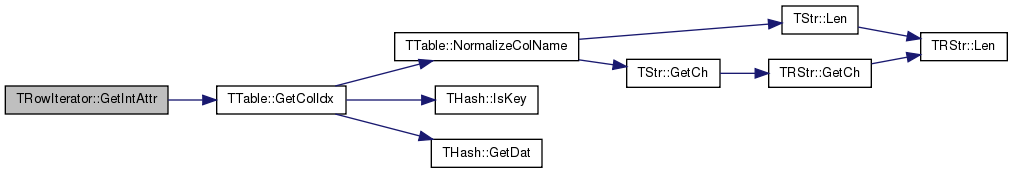TInt TRowIterator::GetRowIdx ( ) const

Gets the id of the row pointed by this iterator.

Definition at line 151 of file table.cpp.

References CurrRowIdx.

151  {
152  return CurrRowIdx;
153 }
TInt CurrRowIdx
Physical row index of current row pointed by iterator.
Definition: table.h:331

Here is the caller graph for this function: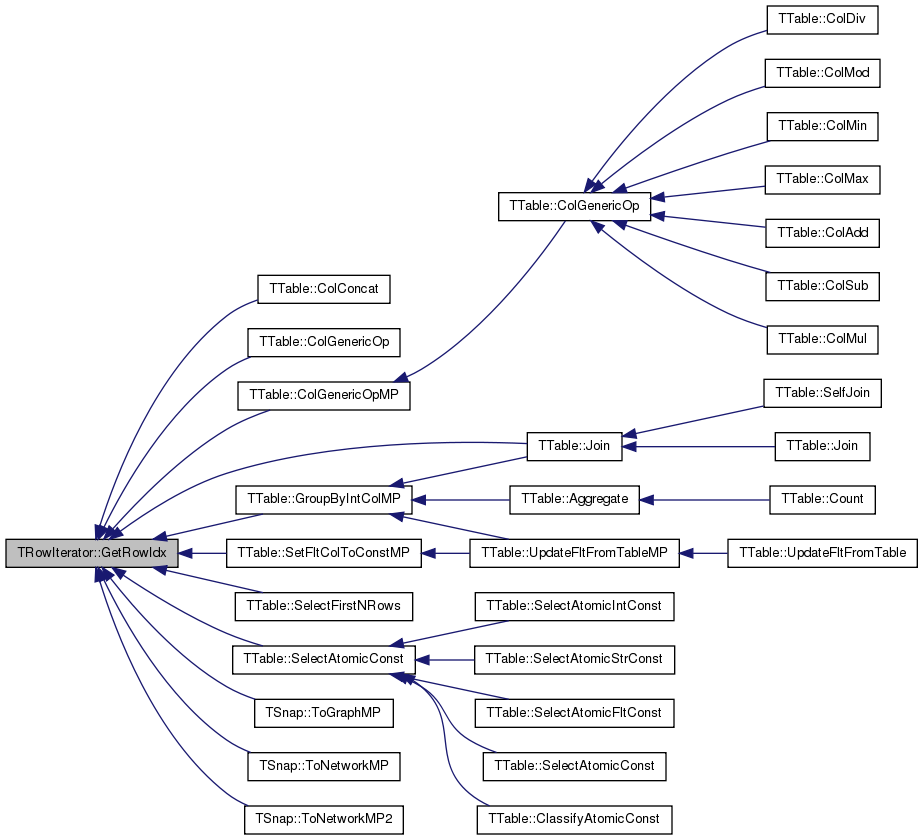TStr TRowIterator::GetStrAttr ( TInt ColIdx ) const

Returns value of string attribute specified by string column index for current row.

Definition at line 163 of file table.cpp.

References CurrRowIdx, TTable::GetStrValIdx(), and Table.

Referenced by TTable::ColConcat(), CompareAtomicConst(), and CompareAtomicConstTStr().

163  {
164  return Table->GetStrValIdx(ColIdx, CurrRowIdx);
165 }
TStr GetStrValIdx(TInt ColIdx, TInt RowIdx) const
Gets the value in column with id ColIdx at row RowIdx.
Definition: table.h:626
const TTable * Table
Reference to table containing this row.
Definition: table.h:332
TInt CurrRowIdx
Physical row index of current row pointed by iterator.
Definition: table.h:331

Here is the call graph for this function: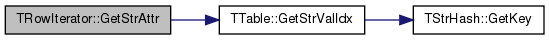Here is the caller graph for this function: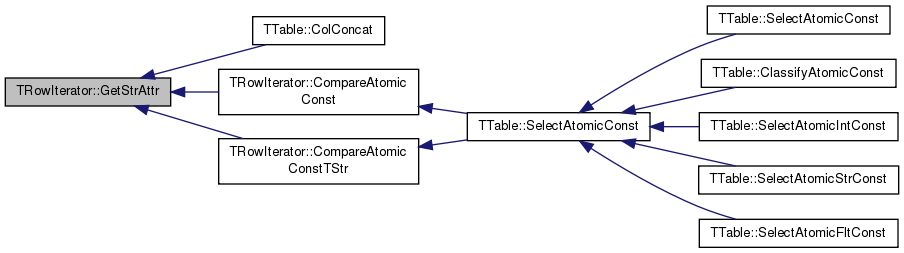TStr TRowIterator::GetStrAttr ( const TStr & Col ) const

Returns value of string attribute specified by attribute name for current row.

Definition at line 177 of file table.cpp.

References CurrRowIdx, TTable::GetStrVal(), and Table.

177  {
178  return Table->GetStrVal(Col, CurrRowIdx);
179 }
const TTable * Table
Reference to table containing this row.
Definition: table.h:332
TStr GetStrVal(const TStr &ColName, const TInt &RowIdx) const
Gets the value of string attribute ColName at row RowIdx.
Definition: table.h:1028
TInt CurrRowIdx
Physical row index of current row pointed by iterator.
Definition: table.h:331

Here is the call graph for this function: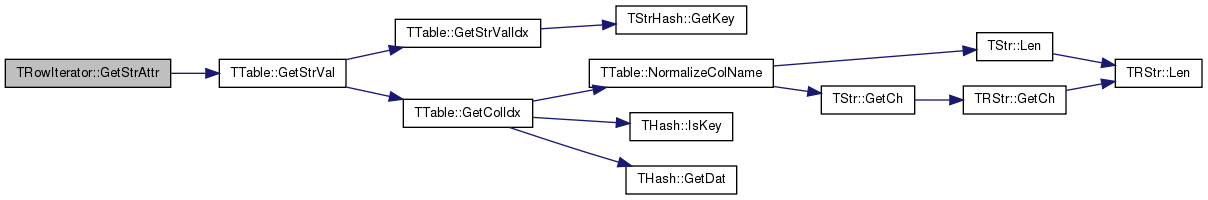TInt TRowIterator::GetStrMapById ( TInt ColIdx ) const

Returns integer mapping of a string attribute value specified by string column index for current row.

Definition at line 186 of file table.cpp.

References CurrRowIdx, TTable::StrColMaps, and Table.

Referenced by TTable::Join(), TSnap::ToGraphMP(), TSnap::ToNetworkMP(), and TSnap::ToNetworkMP2().

186  {
187  return Table->StrColMaps[ColIdx][CurrRowIdx];
188 }
const TTable * Table
Reference to table containing this row.
Definition: table.h:332
TVec< TIntV > StrColMaps
Data columns of integer mappings of string attributes.
Definition: table.h:560
TInt CurrRowIdx
Physical row index of current row pointed by iterator.
Definition: table.h:331

Here is the caller graph for this function: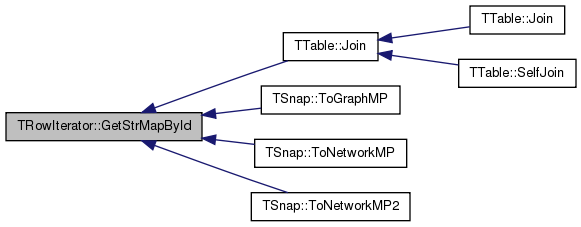TInt TRowIterator::GetStrMapByName ( const TStr & Col ) const

Returns integer mapping of string attribute specified by attribute name for current row.

Definition at line 181 of file table.cpp.

References CurrRowIdx, TTable::GetColIdx(), TTable::StrColMaps, and Table.

181  {
182  TInt ColIdx = Table->GetColIdx(Col);
183  return Table->StrColMaps[ColIdx][CurrRowIdx];
184 }
TInt GetColIdx(const TStr &ColName) const
Gets index of column ColName among columns of the same type in the schema.
Definition: table.h:1013
const TTable * Table
Reference to table containing this row.
Definition: table.h:332
TVec< TIntV > StrColMaps
Data columns of integer mappings of string attributes.
Definition: table.h:560
Definition: dt.h:1137
TInt CurrRowIdx
Physical row index of current row pointed by iterator.
Definition: table.h:331

Here is the call graph for this function: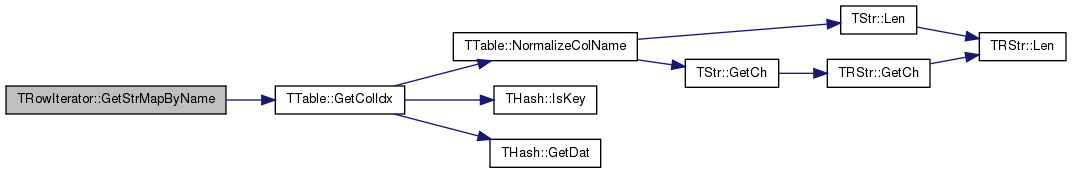Here is the caller graph for this function: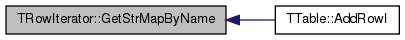TRowIterator & TRowIterator::Next ( )

Increments the iterator (For Python compatibility).

Definition at line 135 of file table.cpp.

References CurrRowIdx, TTable::Next, and Table.

Referenced by operator++().

135  {
137  //Assert(CurrRowIdx != TTable::Invalid);
138  return *this;
139 }
const TTable * Table
Reference to table containing this row.
Definition: table.h:332
TIntV Next
A vector describing the logical order of the rows.
Definition: table.h:555
TInt CurrRowIdx
Physical row index of current row pointed by iterator.
Definition: table.h:331

Here is the caller graph for this function: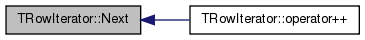TRowIterator & TRowIterator::operator++ ( int )

Increments the iterator.

Definition at line 131 of file table.cpp.

References Next().

131  {
132  return this->Next();
133 }
TRowIterator & Next()
Increments the iterator (For Python compatibility).
Definition: table.cpp:135

Here is the call graph for this function: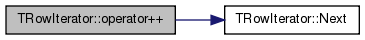bool TRowIterator::operator< ( const TRowIterator & RowI ) const

Checks if this iterator points to a row that is before the one pointed by `RowI`.

Definition at line 141 of file table.cpp.

References CurrRowIdx.

141  {
142  if (CurrRowIdx == TTable::Last) { return false; }
143  if (RowI.CurrRowIdx == TTable::Last) { return true; }
144  return CurrRowIdx < RowI.CurrRowIdx;
145 }
static const TInt Last
Special value for Next vector entry - last row in table.
Definition: table.h:486
TInt CurrRowIdx
Physical row index of current row pointed by iterator.
Definition: table.h:331
 bool TRowIterator::operator== ( const TRowIterator & RowI ) const

Checks if this iterator points to the same row pointed by `RowI`.

Definition at line 147 of file table.cpp.

References CurrRowIdx.

147  {
148  return CurrRowIdx == RowI.CurrRowIdx;
149 }
TInt CurrRowIdx
Physical row index of current row pointed by iterator.
Definition: table.h:331

## Member Data Documentation

 TInt TRowIterator::CurrRowIdx
private

Physical row index of current row pointed by iterator.

Definition at line 331 of file table.h.

 const TTable* TRowIterator::Table
private

Reference to table containing this row.

Definition at line 332 of file table.h.

Referenced by GetFltAttr(), GetIntAttr(), GetStrAttr(), GetStrMapById(), GetStrMapByName(), and Next().

The documentation for this class was generated from the following files: